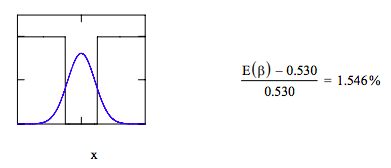Skip to main content

# 421: Variational Method for a Particle in a Finite Potential Well

Definite potential energy: $$V(x) := if [(x \geq -1) \cdot (x \leq 1), 0, 2]$$

Display potential energy:Choose trial wavefunction: $$\psi (x, \beta ) := ( \frac{2 \cdot \beta}{ \pi} )^{ \frac{1}{4}} \cdot (- \beta \cdot x^{2})$$

Demonstrate that the trial wavefunction is normalized.

$$\int_{- \infty}^{ \infty} \psi (x, \beta )^{2} dx$$ assume, $$\beta > 0 \rightarrow 1$$

Evaluate the variational integral:

$$E( \beta ) := \int_{- \infty}^{ \infty} \psi (x, \beta ) \cdot - \frac{1}{2} \cdot \frac{d^{2}}{dx^{2}} \psi (x, \beta ) dx ... |_{simplify}^{assume,~ \beta > 0} \rightarrow \frac{1}{2} \cdot \beta + 2 - 2 \cdot erf(2^{ \frac{1}{2}} \cdot \beta^{ \frac{1}{2}})$$

Minimize the energy integral with respect to the variational parameter, $$\beta$$.

$$\beta$$ := 1 $$\beta$$ := Minimize(E, $$\beta$$) $$\beta$$ = 0.678 E( $$\beta$$) = 0.538

Display wavefunction in the potential well and compare result with the exact energy, 0.530 Eh.Calculate the fraction of time tunneling is occurring.

$$2 \cdot \int_{1}^{ \infty} \psi (x, \beta )^{2} dx = 0.1$$

• Was this article helpful?